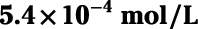##### Chemistry For DummiesIncreasing temperature magnifies the effects of entropy on a system. Because the entropy of a solute is usually increased when it dissolves, increasing temperature usually increases solubility — for solid and liquid solutes, anyway. Another way to understand the effect of temperature on solubility is to think about heat as a reactant in the dissolution reaction: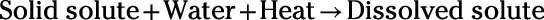Heat is usually absorbed into solute particles when a solute dissolves. Increasing temperature corresponds to added heat. So by increasing temperature, you supply a needed reactant in the dissolution reaction. (In those rare cases where dissolution releases heat, increasing temperature can decrease solubility.)

Gaseous solutes behave differently from solid or liquid solutes with respect to temperature. Increasing the temperature tends to decrease the solubility of gas in liquid. To understand this pattern, check out the concept of vapor pressure. Increasing temperature increases vapor pressure because added heat increases the kinetic energy of the particles in solution. With added energy, these particles stand a greater chance of breaking free from the intermolecular forces that hold them in solution.

A classic, real-life example of temperature's effect on gas solubility is carbonated soda. Which goes flat (loses its dissolved carbon dioxide gas) more quickly — warm soda or cold soda?

Comparing gas solubility in liquids with the concept of vapor pressure highlights another important pattern: Increasing pressure increases the solubility of a gas in liquid. Just as high pressures make it more difficult for surface-dwelling liquid molecules to escape into vapor phase, high pressures inhibit the escape of gases dissolved in solvent. Henry's law summarizes this relationship between pressure and gas solubility:

Solubility = Constant x Pressure
The "constant" in Henry's law is Henry's constant, and its value depends on the gas, solvent, and temperature. A particularly useful form of Henry's law relates the solubility (S) and pressure (P) between two different states: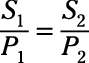According to this relationship, tripling the pressure triples the gas solubility.

Try an example. Henry's constant for nitrogen gas (N2) in water at 293 Kelvin (K) is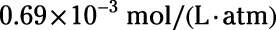The partial pressure of nitrogen in air at sea level is 0.78 atm. What is the solubility of N2 in a glass of water at 20 degrees Celsius sitting on a coffee table within a beach house?

This problem requires the direct application of Henry's law. The glass of water is at 20 degrees Celsius, which is equivalent to 293 K (just add 273 to any Celsius temperature to get the Kelvin equivalent). Because the glass sits within a beach house, you can assume the glass is at sea level. So you can use the provided values for Henry's constant and the partial pressure of N2: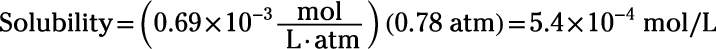So, the solubility of N2 is